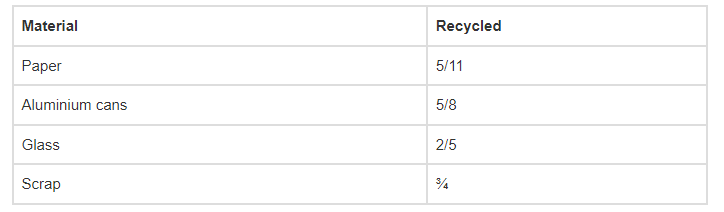# The table shows the portion of some

Question:

The table shows the portion of some common materials that are recycled.(a) Is the rational number expressing the amount of paper recycled more than ½ or less than ½?

(b) Which items have a recycled amount less than ½?

(c) Is the quantity of aluminium cans recycled more (or less) than half of the quantity of aluminium cans?

(d) Arrange the rate of recycling the materials from the greatest to the smallest.

Solution:

(a) Is the rational number expressing the amount of paper recycled more than ½ or less than ½?

The rational number expressing the amount of paper recycled is less than ½.

(b) Which items have a recycled amount less than ½?

Paper and Glass have a recycled amount less than ½.

(c) Is the quantity of aluminium cans recycled more (or less) than half of the quantity of aluminium cans?

The quantity of aluminium cans recycled is more than half of the quantity of aluminium cans.

(d) Arrange the rate of recycling the materials from the greatest to the smallest.

The LCM of the denominators 11, 8, 5 and 4 is 440

∴ 5/11 = [(5×40)/ (11×40)] = (200/440)

(5/8) = [(5×55)/ (8×55)] = (275/440)

(2/5) = [(2×88)/ (5×88)] = (176/440)

(3/4) = [(3×110)/ (4×110)] = (330/440)

Then,

Now, 330 > 275 > 200 > 176

Hence, ⇒ 3/4 > 5/8 > 5/11 > 2/5

∴Scrap > Aluminium > Cans > Paper > Glass.